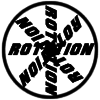#### You may also like### 8 Methods for Three by One

This problem in geometry has been solved in no less than EIGHT ways by a pair of students. How would you solve it? How many of their solutions can you follow? How are they the same or different? Which do you like best?### Rots and Refs

Follow hints using a little coordinate geometry, plane geometry and trig to see how matrices are used to work on transformations of the plane.### Reflect Again

Follow hints to investigate the matrix which gives a reflection of the plane in the line y=tanx. Show that the combination of two reflections in intersecting lines is a rotation.

# Square Pair

##### Age 16 to 18 Challenge Level:
Try transforming the points of $S$ using some different $2 \times 2$ matrices and record the resulting points on a grid. What do you notice?

This problem uses mathematical ideas met in Further Pure Mathematics A Level modules.